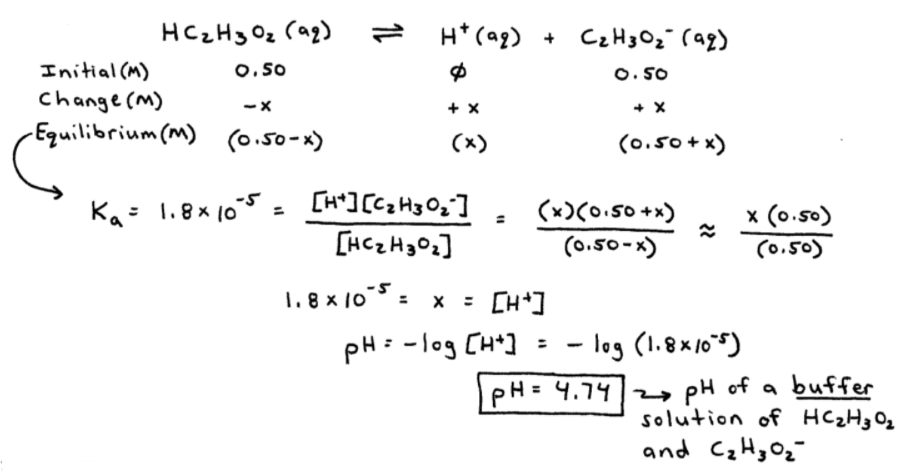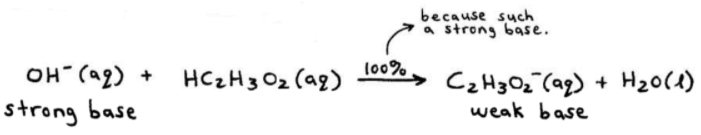# S15E2 - Buffers and Buffer Solution pH Calculations

## Buffer Solutions and pH Calculations.

Buffer problems in Chemistry can be quite complex, so it's always good to have a plan -- a map of "how you're going to solve" the problem.

Let's dive in with our first example...

ex:  A buffer solution contains 0.50M acetic acid (Ka = 1.8 x 10-5) and 0.50M sodium acetate. Calculate the pH of this solution.

➞ identify the major species first:  HC2H3O2,  Na+ (neutral),  C2H3O2-,  H2O (neutral)

➞ so we have our ICE Table:Now, because this is a [HC2H3O2  /  C2H3O2-] buffer solution, or "buffer," it resists a change in its pH upon the addition of acid (H+) or base (OH-).

-----

For example, what would happen if we added some strong base (i.e. NaOH) to the pH 4.74 buffer in the above problem?

Let's see...

ex:  Calculate the change in pH that occurs when 0.010 mol NaOH(s) is added to 1.0L of the buffer prepared in the last example. Then, compare this pH change with that which occurs when 0.010 mol NaOH(s) is added to 1.0L of water.

the approach:

1. realize that NaOH(s) completely dissociates and the OH- reacts completely (100%) with any acid that's around.

-  Carry out these stoichiometric calculations.

2. do the equilibrium problem (ICE Table, or ICE Method).

---

➞ here we go:

1. major species:   Na+ (neutral),   OH-,   HC2H3O2,   C2H3O2-,   H2O (neutral)

OH- is a strong base, so it will "grab" all the H+ that it can:2. now, the equilibrium problem (after all OH- has reacted with HC2H3O2).Wow, we added a very strong base (NaOH) to our original buffer solution, but the change in pH was minimal: 4.76 - 4.74 = 0.02 pH units.

This is what buffers do!

They resist large changes to their pH upon the addition of acids or bases.

But WAIT -- we're not done with the problem.

We were also asked to calculate the pH change if the strong base (NaOH) were added to pH = 7.00 water...=====

## Question: How Exactly Does a Buffer Work?

Why does its pH not increase substantially when a strong base (i.e. NaOH) is added?

Answer:  The net result is that free OH- ions are not allowed to accumulate.

For example, OH-(aq) in the last sample problem is not around for very long, but instead it gets replaced by the much weaker base, C2H3O2- , according to:NOTE
-- the same is true when a strong acid (H+) such as HCl is added to a buffer.

The strong acid (HCl) is replaced by a much weaker acid (perhaps NH4+), and the pH will only decrease by a small amount.

=====

Next up in our discussion of SECTION 15 - Applications of Acid-Base Equilibria,

We'll talk about the Henderson-Hasselbalch Equation and of course, more buffers...